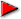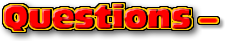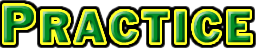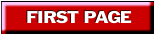ChaptersIntroduction Page 1This text is meant to accompany class discussions. It is not everything there is to know about dimensional analysis. It is meant as a  prep for class. More detailed notes and examples are given in the class notes, presentations, and demonstrations (click here.)Click for the questions that go with this reading

Introduction

In medieval times, an team of ox could plow a furrow in a day. How long is this furrow as measured in meters?

To do this you would use a technique called dimensional analysis. (Sometime this system is also called the "factor label" system.) This technique uses the units or dimensions of the numbers to create a math relationship that will allow you to switch between units. This is done by changing equations to fractions.

If you had the following setup, how would your math teacher show you to solve it?

An equality describes two concepts as being equal. The statement, "5280 feet = 1609 meters," is an equality. The "Fact Sheet" lists many equalities that will be used in class. This particular fact sheet does not show all the equalities. It shows a collection of equalities that will use most often in this course. (Note there are a few equalities you need to memorize for this course. These equalities will not be appear on any fact sheet passed out for your tests or quizzes.) Equalities can be written as fractions. For example.

This is a useful tool. This means that any unit equality can be written as a fraction. Back to the original question, "In medieval times a team of ox could plow a furrow in a day. How long is this furrow as measured in meters?"

The video above can be found on YouTube at http://www.youtube.com/watch?v=xUa3ORM8xjE• Question
• Numerical Answer
• Solution
• Your Paper
It takes a dog 2 hours to wander 17 football fields away from home. What is the dog's displacement in meters? (Work out this problem and check your answer)
 The answer is 1554.48 m

All fractions are created using the equalities from the fact sheet. In order to solve this you will have to switch units systems. The conversion to remember when switching between the British customary system and the S.I. system is

2.54 cm = 1 inch

Start the set up with the distance you are given, 17 football fields, and convert to inches then cross over to the metric system.To solve this on a graphing calculator you would put all the number in numerator in parenthesis and divide it by all the numbers in the demonstrator in parenthesis. Your graphing calculator screen would look something like this:This is what your answer should look like. Notice how number has a unit next to it and the answer is in a box.• Question 2
• Numerical Answer
• Solution
• Your Paper
A large molar has a mass of 3.5 grams. How many "grains" is the weight of the molar? Before checking out the answer, do the calculation.
 54.01 grains

All fractions come from the fact sheet.Your graphing calculator would look something like this:This is a very basic dimensional analysis. In class we will discuss conversion of fractional units and units raised to a power. Click here for the class notes.by Tony Wayne ...(If you are a teacher, please feel free to use these resources in your teaching.)